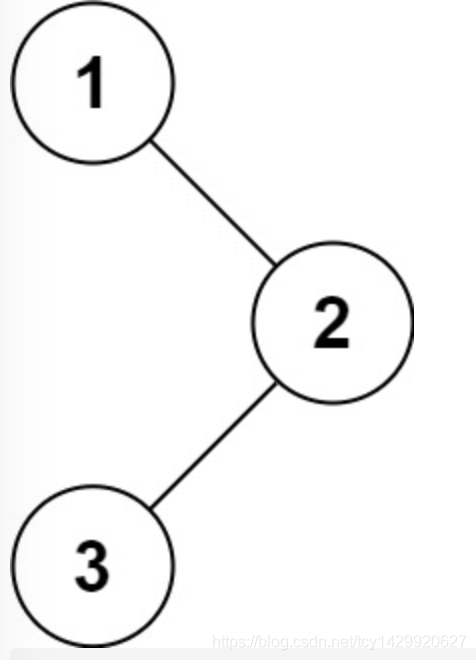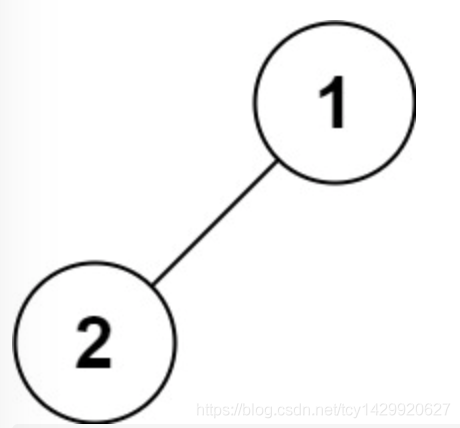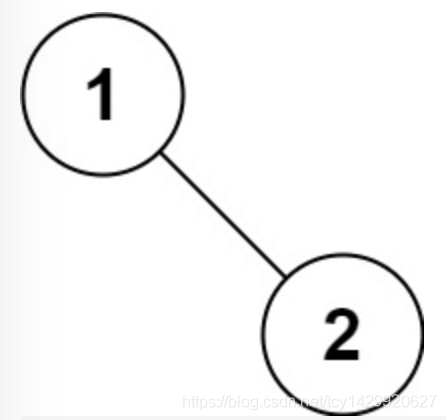# Title Description

Give you the root node of the binary tree root , Of its node value   Before the order   Traverse .

Example 1：Input ：root = [1,null,2,3]
Output ：[1,2,3]
Example 2：

Input ：root = []
Output ：[]
Example 3：

Input ：root = 
Output ：
Example 4：Input ：root = [1,2]
Output ：[1,2]
Example 5：Input ：root = [1,null,2]
Output ：[1,2]

Tips ：

The number of nodes in the tree is in the range [0, 100] Inside
-100 <= Node.val <= 100

source ： Power button （LeetCode） link ：https://leetcode-cn.com/problems/binary-tree-preorder-traversal

# Their thinking

First of all, we need to know what is preorder traversal of a binary tree ： Access the root node according to —— The left subtree —— The right subtree traverses the tree , And when you visit the left or right subtree , We traverse in the same way , Until you walk through the whole tree . Therefore, the whole traversal process has the nature of recursion , We can directly use recursive functions to simulate this process .

Definition preorder(root) Indicates that the current traversal to root The answer to the node . By definition , We just need to first root The value of the node is added to the answer , Then recursively call preorder(root.left) To traverse root The left subtree of the node , Finally, recursively call preorder(root.right) To traverse root The right subtree of the node can be , The condition for the termination of recursion is to hit an empty node .

# Solution code

Complexity analysis

Time complexity ：O(n), among n It's the number of nodes in a binary tree . Each node is traversed exactly once .

Spatial complexity ：O(n), For the stack overhead in recursion , On average O(logn), At worst, the tree is chained , by O(n).

``````/**
* Definition for a binary tree node.
* public class TreeNode {
*     int val;
*     TreeNode left;
*     TreeNode right;
*     TreeNode() {}
*     TreeNode(int val) { this.val = val; }
*     TreeNode(int val, TreeNode left, TreeNode right) {
*         this.val = val;
*         this.left = left;
*         this.right = right;
*     }
* }
*/
class Solution {
List<Integer> res = new ArrayList<>();
public List<Integer> preorderTraversal(TreeNode root) {
preorder(root);
return res;
}
public void preorder(TreeNode root) {
if(root == null) {
return;
}